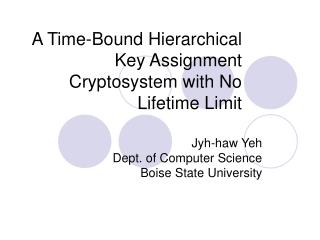DownloadDownload PresentationA Time-Bound Hierarchical Key Assignment Cryptosystem with No Lifetime Limit

# A Time-Bound Hierarchical Key Assignment Cryptosystem with No Lifetime Limit

Télécharger la présentation## A Time-Bound Hierarchical Key Assignment Cryptosystem with No Lifetime Limit

- - - - - - - - - - - - - - - - - - - - - - - - - - - E N D - - - - - - - - - - - - - - - - - - - - - - - - - - -
##### Presentation Transcript

1. A Time-Bound Hierarchical Key Assignment Cryptosystem with No Lifetime Limit Jyh-haw Yeh Dept. of Computer Science Boise State University

2. Problem history • The problem was first proposed by Tzeng (2002). • Alternative schemes: Chien’s (2004), Huang and Chang’s (2004), Wang and Laih’s (2006). • Tzeng’s, Chien’s, Huang and Chang’s schemes were broken. • Wang and Laih’s scheme is secure but cannot assign keys on the fly.

3. Problem • A system is divided into n classes. • These n classes form a hierarchical structure. • Time is divided into periods. • Each user is assigned to a class C_i with a set of time periods T. • The system give the user a user key K_{I,T}.

4. Problem (cont…) • Each class C_j in each time period t encrypts its own data by a key K_{j,t}. • A user, in C_i with time periods T, is able to access data in C_j encrypted at time t iff C_i is higher than C_j and t is in T. • The problem becomes assigning keys so that K_{i,T}  K_{j,t} iff C_i is higher than C_j and t is in T.

5. Problem (cont…)

6. Application • A publisher publishes journals periodically. • Different packages of journals for subscription. • Users subscribe packages for different time periods. • Only subscribed users can access the journals published in the time periods the users subscribed to.

7. Application (cont…) • The journals can be just posted online, but encrypted. • Each journal published in different time period is encrypted by a different key . • Each user has one user key. • The user key can decrypt only the journals the user are entitled to access.

8. An Example

9. Key Assignment • Based on RSA algorithm. • 4 processes: • Initialization: compute parameters. • Encryption key Generation: assign an encryption key for each class at each time period. • User key generation: • Key derivation: use a user key and some public parameters to derive an encryption.

10. Key assignment (cont…)

11. Key Assignment (cont…)

12. Key Assignment (cont…)

13. Key Assignment (cont…)

14. Comparison

15. Future Works • Develop dynamic management component of the scheme: add (delete) new classes, add (delete) users, add (delete) time periods to (from) users. • Comprehensive analysis of the efficiency of the scheme: storage, computation… • Formal proofs of the security.